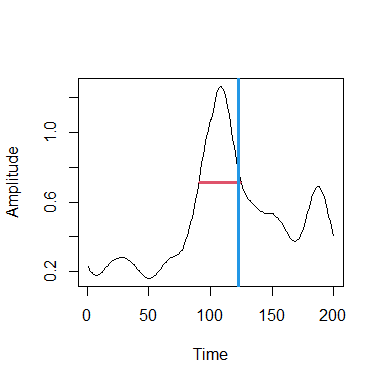# Muscle synergy analysis with musclesyneRgies

library(musclesyneRgies)

## Analysis functions

For example, one can measure the full width at half maximum (FWHM) of the motor primitives or their centre of activity (CoA).

# Load a typical motor primitive of 30 cycles (from locomotion)
data("primitive")

# Reduce primitive to the first cycle
prim_sub <- primitive$signal[1:which(primitive$time == max(primitive$time))] # Calculate FWHM of the first cycle prim_sub_FWHM <- FWHM(prim_sub) # Calculate CoA of the first cycle prim_sub_CoA <- CoA(prim_sub) # Half maximum (for the plots) hm <- min(prim_sub) + (max(prim_sub) - min(prim_sub)) / 2 hm_plot <- prim_sub hm_plot[which(hm_plot > hm)] <- hm hm_plot[which(hm_plot < hm)] <- NA # Plots plot(prim_sub, ty = "l", xlab = "Time", ylab = "Amplitude") lines(hm_plot, lwd = 3, col = 2) # FWHM (horizontal, in red) graphics::abline(v = prim_sub_CoA, lwd = 3, col = 4) # Coa (vertical, in blue)Or perhaps one might want to investigate the nonlinear behaviour of a long primitive. prim <- primitive$signal

# Calculate the local complexity or Higuchi's fractal dimension (HFD)
nonlin_HFD <- HFD(prim)$Higuchi # Calculate the local complexity or Hurst exponent (H) nonlin_H <- Hurst(prim, min_win = max(primitive$time))\$Hurst

message("Higuchi's fractal dimension: ", round(nonlin_HFD, 3))
#> Higuchi's fractal dimension: 1.047
message("Hurst exponent: ", round(nonlin_H, 3))
#> Hurst exponent: 0.338